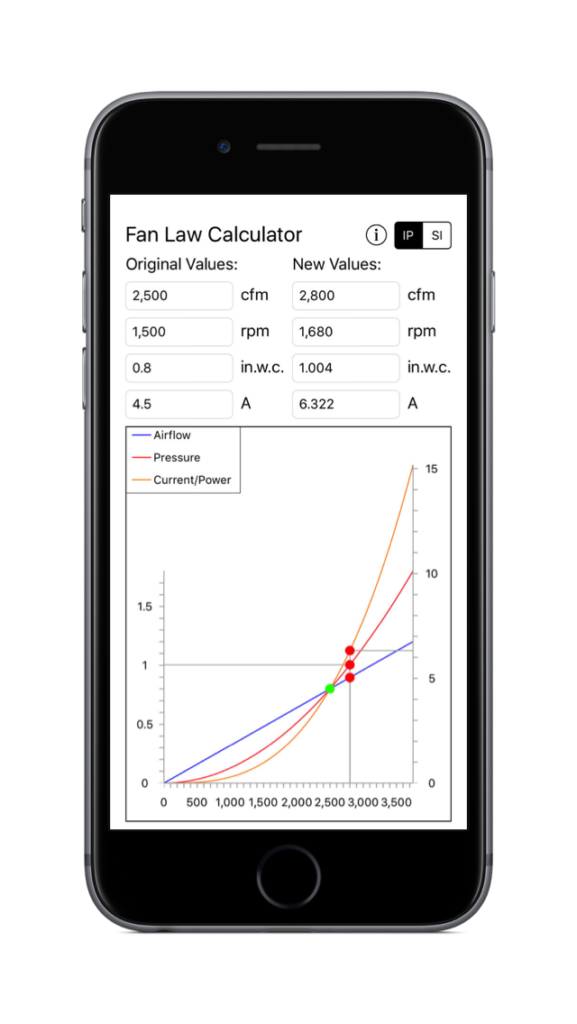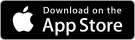Fan Law Calculator

Fan Law Calculator

The Fan Law Calculator for iPhone/iPad has been designed to allow quick estimation of the performance impact of variation in airflow and/or rpm on an existing system. The standard fan law equations are used to estimate the system performance changes.

Usage

Input Original Values

Input the current values for airflow, rpm, pressure and current (or power) into the appropriate text field. The calculations will automatically update as you type.

Input Updated Values For Performance Estimation

The updated values can be input in one of two ways. The new values can be entered into the appropriate text field. Or, you can drag the airflow value left and right on the graph view to change the values. The calculations will automatically update as you edit the values.

Calculations

The standard fan law equations are applied:

cfm2 = cfm1 * rpm2/rpm1

sp2 = sp1 * (cfm2/cfm1)^2

power2 = power1 * (cfm2/cfm1)^3